Next: B.2 Selected FCS Power Up: B. Conversion Algorithms for Previous: B. Conversion Algorithms for

Subsections

# B.1 Temperature Sensors

### Detector temperature

To convert ERD voltage to actual detector temperature one should apply the following relationship: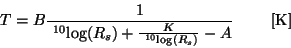(B.1)

where:


A, B, K = constants, different for each sensor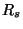= sensor resistance in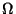, according to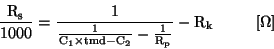(B.2)

Where:


tmd = telemetry data value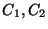= constants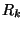= harness resistance in k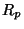= EEU shunt resistor in parallel to sensor in kSensor A B K FCS1 6.54 2.90 10.67 FCS2 6.54 2.90 10.67 C100 5.44 3.05 7.34 C200 4.51 3.12 4.92 P1 6.02 3.61 8.77 P2 7.59 3.13 14.40 P3 5.11 3.49 6.29 S1 6.54 2.94 10.66 S2 6.54 2.90 10.67

 Sensor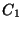[k]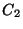[k][k][k] FCS1 0.259 495.83 1.12 1000 FCS2 0.256 495.83 1.12 1000 C100 0.099 146.38 1.34 infinite C200 0.048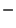78.32 0.67 infinite P1 0.174 344.55 1.12 499 P2 0.210 388.13 1.34 499 P3 0.182 288.11 1.34 infinite S1 0.173 341.63 1.12 499 S2 0.170 336.88 1.12 499

### EEU temperature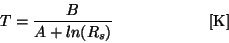(B.3)

with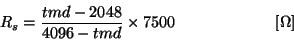(B.4)

where


A =	3.9489
B =	3442 K
R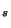=    sensor resistance inT =	EEU Temperature in K
tmd =	telemetry data valueNext: B.2 Selected FCS Power Up: B. Conversion Algorithms for Previous: B. Conversion Algorithms for
ISO Handbook Volume IV (PHT), Version 2.0.1, SAI/1999-069/Dc